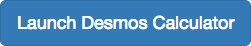## Compound and Absolute Value Inequalities

### Learning Outcomes

• Solve compound inequalities.
• Solve absolute value inequalities.

A compound inequality includes two inequalities in one statement. A statement such as $4<x\le 6$ means $4<x$ and $x\le 6$. There are two ways to solve compound inequalities: separating them into two separate inequalities or leaving the compound inequality intact and performing operations on all three parts at the same time. We will illustrate both methods.

### Example: Solving a Compound Inequality

Solve the compound inequality: $3\le 2x+2<6$.

### Try It

Solve the compound inequality $4<2x - 8\le 10$.

### Example: Solving a Compound Inequality with the Variable in All Three Parts

Solve the compound inequality with variables in all three parts: $3+x>7x - 2>5x - 10$.

### Try It

Solve the compound inequality: $3y<4 - 5y<5+3y$.

## Solving Absolute Value Inequalities

As we know, the absolute value of a quantity is a positive number or zero. From the origin, a point located at $\left(-x,0\right)$ has an absolute value of $x$ as it is x units away. Consider absolute value as the distance from one point to another point. Regardless of direction, positive or negative, the distance between the two points is represented as a positive number or zero.

An absolute value inequality is an equation of the form

$|A|<B,|A|\le B,|A|>B,\text{or }|A|\ge B$,

where A, and sometimes B, represents an algebraic expression dependent on a variable x. Solving the inequality means finding the set of all $x$ values that satisfy the problem. Usually this set will be an interval or the union of two intervals and will include a range of values.

There are two basic approaches to solving absolute value inequalities: graphical and algebraic. The advantage of the graphical approach is we can read the solution by interpreting the graphs of two equations. The advantage of the algebraic approach is that solutions are exact, as precise solutions are sometimes difficult to read from a graph.

Suppose we want to know all possible returns on an investment if we could earn some amount of money within $200 of$600. We can solve algebraically for the set of x-values such that the distance between $x$ and 600 is less than 200. We represent the distance between $x$ and 600 as $|x - 600|$, and therefore, $|x - 600|\le 200$ or

$\begin{array}{c}-200\le x - 600\le 200\\ -200+600\le x - 600+600\le 200+600\\ 400\le x\le 800\end{array}$

This means our returns would be between $400 and$800.

To solve absolute value inequalities, just as with absolute value equations, we write two inequalities and then solve them independently.

### A General Note: Absolute Value Inequalities

For an algebraic expression and $k>0$, an absolute value inequality is an inequality of the form:

$\begin{array}{l}|X|< k\text{ which is equivalent to }-k< X< k\hfill \text{ or }\ |X|> k\text{ which is equivalent to }X< -k\text{ or }X> k\hfill \end{array}$

These statements also apply to $|X|\le k$ and $|X|\ge k$.

### Example: Determining a Number within a Prescribed Distance

Describe all values $x$ within a distance of 4 from the number 5.

### Try It

Describe all x-values within a distance of 3 from the number 2.

### Example: Solving an Absolute Value Inequality

Solve $|x - 1|\le 3$.

### Example: Using a Graphical Approach to Solve Absolute Value Inequalities

Given the equation $y=-\frac{1}{2}|4x - 5|+3$, determine the x-values for which the y-values are negative.

### Try It

Solve $-2|k - 4|\le -6$.

### Try It

Sometimes a picture is worth a thousand words. You can turn a single variable inequality into a two variable inequality and make a graph. The x-intercepts of the graph will correspond with the solution to the inequality you can find by hand.
Let’s use the last example to try it. We will change the variable to x to make it easier to enter in Desmos.
To turn $-2|x - 4|\le -6$ into a two variable equation, move everything to one side, and place the variable y on the other side like this:

$-2|x - 4|\le -6$
$-2|x - 4|+6\le y$

Now enter this inequality in Desmos and hover over the x-intercepts.If you need instruction on how to enter inequalities in Desmos, watch this tutorial.

Are the x-values of the intercepts the same values as the solution we found above?

Now you try turning this single variable inequality into a two variable inequality:

$5|9-2x|\ge10$

Graph your inequality with an online graphing tool, and write the solution interval.

## Contribute!

Did you have an idea for improving this content? We’d love your input.